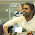## Sunday, December 28, 2014

### Sorting a given stack using one additional stack.

Q. Write a program to sort a stack in ascending order (with biggest items on top). You may use at most one additional stack to hold items, but you may not copy the elements into any other data structure (such as an array). The stack supports the following operations: push, pop, peek and isEmpty.

Algorithm:

1. We will have two stacks, first one is the given unsorted one and the second one will be sorted which will be returned by the method call.
2. Pop an item from first stack and push it to the second.
3. Pop an item from the unsorted (first) stack (storing it in a temp variable) and compare it with sorted array's head. If head is larger, pop it and push it to unsorted stack. Do this until the value at head is < or = to the temp element.
4. At the time when the loop stops, the value below is less than or equal to temp variable, we can now push it to the sorted stack.
5. Now push back all the items that were originally in stack 2 and were shifted to stack 1 to accommodate the temp item.
6. Do this until stack 1 becomes empty.
7. Stack 2 contains the sorted data and can now be returned.

Source:

```class OrderedStack<T extends Comparable<T>> extends Stack<T>{
public OrderedStack(int size) {
super(0);
elements = (T[])new Comparable[size];
}
}

class StackSorter{

public static <T extends Comparable<T>> OrderedStack<T> sort(Stack<T> unsorted){
OrderedStack<T> sorted = new OrderedStack<>(unsorted.size());
if(unsorted.isEmpty())return sorted;

sorted.push(unsorted.pop());
while(!unsorted.isEmpty()){
T temp = unsorted.pop();
int i=0;
while(!sorted.isEmpty() && sorted.peek().compareTo(temp)>0){
unsorted.push(sorted.pop());i++;
}
sorted.push(temp);
while(i--!=0)sorted.push(unsorted.pop());
}
return sorted;
}
}

public class Code{
public static void main(String[] args){
Integer test[] = {2,3,4,4,2,22,34,5,6,7,8,867,1,5};
Stack<Integer> stack = new Stack<>(test.length);

for(int i:test)stack.push(i);
stack = StackSorter.sort(stack);
while(!stack.isEmpty())System.out.print(stack.pop()+ " ");
}
}

public class Stack <T>{
int top = -1;
T[] elements;

public Stack(int size){
elements = (T[])new Object[size];
}

public int size(){
}

public void push(T element){
if(top+1 == elements.length){
System.out.println("Overflow.");
return;
}
elements[++top] = element;
}

public T pop(){
if(top<0){
System.out.println("Underflow.");
return null;
}
return elements[top--];
}

public T peek(){
if(top < 0){
System.out.println("Stack is empty!");
return null;
}
return elements[top];
}

public boolean isEmpty(){
1.